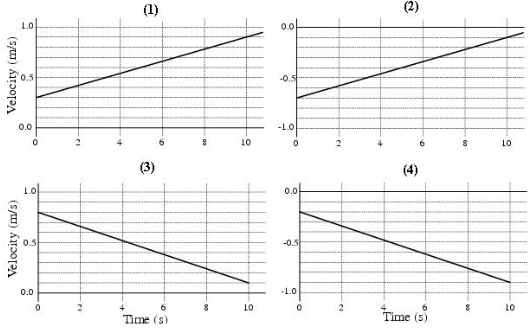# Problem: The following three questions are related to the four velocity versus time graphs (1-4) given in the figure. The answer to each question is two of the four graphs. a) Which graphs represent an object moving in the positive direction?b) Which graphs represent an object that is speeding up?c)Which graphs represent an object that has a positive acceleration?

###### FREE Expert Solution

(a) An object moves in the positive direction if the velocity is positive. Velocity is positive for graphs 1 and 3.

Graphs and 3 represent an object moving in the positive direction.

94% (129 ratings)###### Problem Details

The following three questions are related to the four velocity versus time graphs (1-4) given in the figure. The answer to each question is two of the four graphs.

a) Which graphs represent an object moving in the positive direction?

b) Which graphs represent an object that is speeding up?

c)Which graphs represent an object that has a positive acceleration?